15.2.3 Trigonometry, PT3 Focus Practice

Question 10:
A technician needs to climb a ladder to repair a street lamp as shown in Diagram below.(a) What is the length, in m, of the ladder?
(b) Find the height, in m, of the lamp post.

Solution: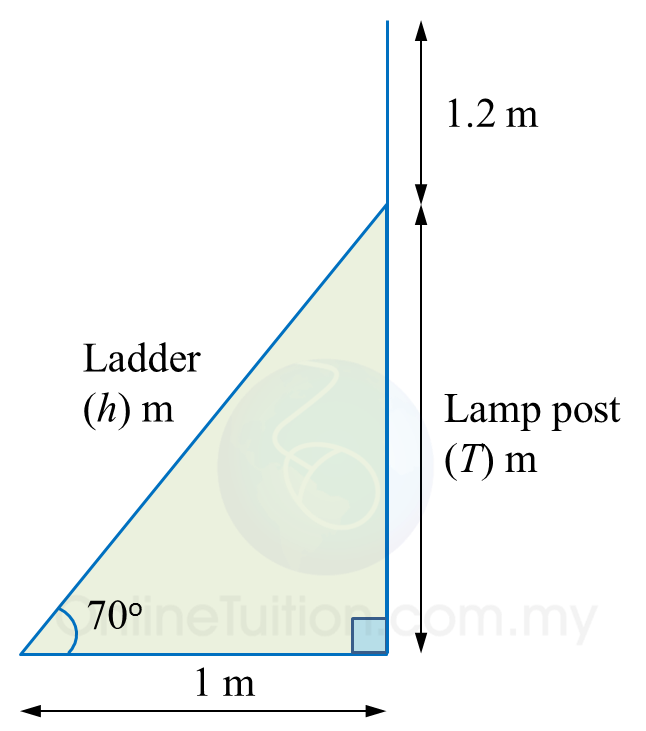(a)

(b)

15.2.2 Trigonometry, PT3 Focus Practice

Question 6:
In diagram below, AEC and BCD are straight lines. E is the midpoint of AC.(a) find the value of tan x.
(b) Calculate the length, in cm, of BD.

Solution:

Question 7:
In diagram below, T is the midpoint of the line PR.(a) Find the value of tan xo.
(b) Calculate the length, in cm, of PQ.

Solution:

Question 8:
In diagram below, ABE and DBC are two right-angled triangles ABC and DEB are straight lines.(a) Find the value of tan xo.
(b) Calculate the length, in cm, of DE.

Solution:

Question 9:
Diagram below shows a vertical pole, PQ. At 2.30 p.m. and 5.00 p.m., the shadow of the pole falls on QR and QS respectively.Calculate
(a) the height, in m, of the pole.
(b) the value of w.

Solution:
(a)

(b)

15.2.1 Trigonometry, PT3 Focus Practice

15.2.1 Trigonometry, PT3 Focus Practice

Question 1:
Diagram below shows a right-angled triangle ABC.It is given that  $\mathrm{cos}{x}^{o}=\frac{5}{13}$ , calculate the length, in cm, of AB.

Solution:

Question 2:
In the diagram, PQR and QTS are straight lines.It is given that $\mathrm{tan}y=\frac{3}{4}$ , calculate the length, in cm, of RS.

Solution:

Question 3:
In the diagram, PQR is a straight line.It is given that   $\mathrm{cos}{x}^{o}=\frac{3}{5}$ , hence sin yo =

Solution:

Question 4:
Diagram below consists of two right-angled triangles.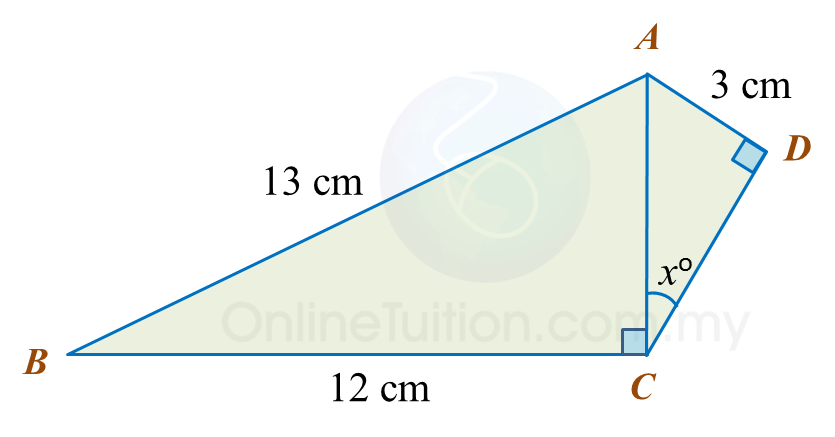Determine the value of cos xo.

Solution:

Question 5:
Diagram below consists of two right-angled triangles ABE and DBC.
ABC and EBD are straight lines.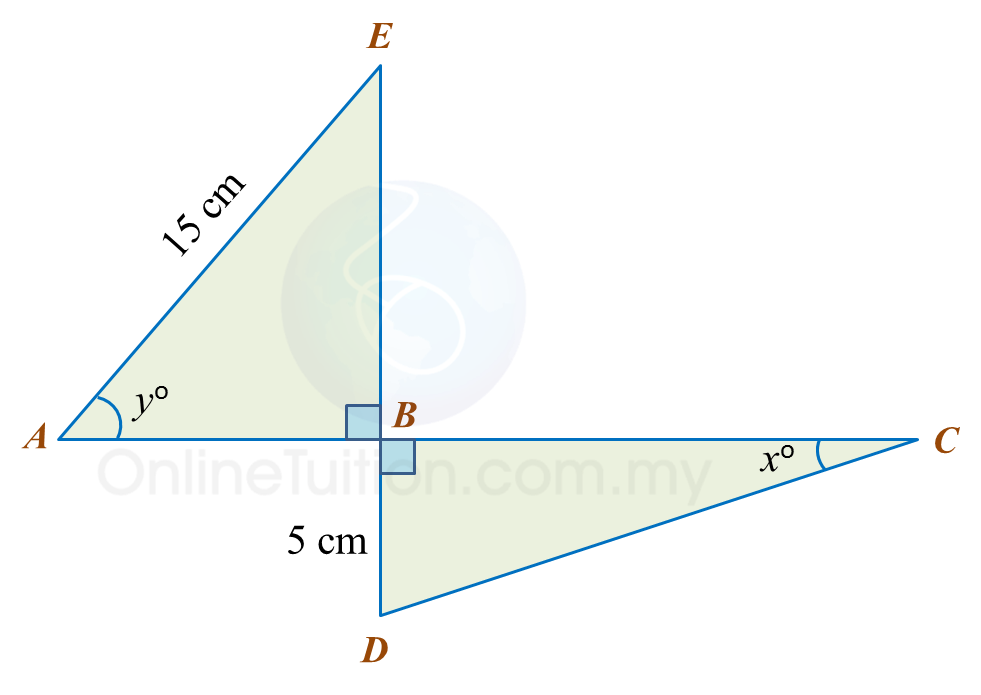It is given that
(a) Find the value of tan xo.
(b)   Calculate the length, in cm, of ABC.

Solution:
(a)

(b)

15.1 Trigonometry

15.1 Trigonometry

15.1.1 Trigonometrical Ratios of an Acute Angle

1.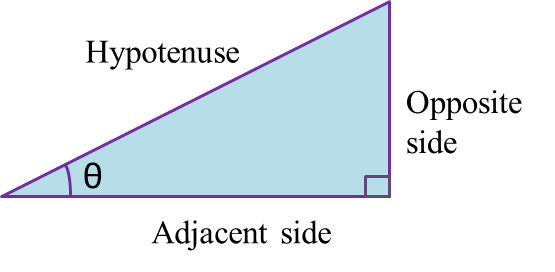2.   Hypotenuse is the longest side of the right-angled triangle which is opposite the right angle.
3.   Adjacent side is the side, other than the hypotenuse, which has direct contact with the given angle, θ.
4.   Opposite side is the side which is opposite the given angle, θ.
5.   In a right-angled triangle,

6.
When the size of an angle θ increases from 0o to 90o,

· sin θ increases.
· cos θ increases.
· tan θ increases.

7.   The values of sin θ, cos θ and tan θ remain the same even though the size of the triangle has changed.
Example: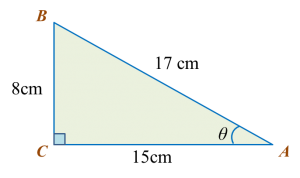Find the sine, cosine and tangent of the give angle, θ, in triangle ABC.

Solution:
$\begin{array}{l}\mathrm{sin}\theta =\frac{BC}{AB}=\frac{8}{17}\\ \mathrm{cos}\theta =\frac{AC}{AB}=\frac{15}{17}\\ tan\theta =\frac{BC}{AC}=\frac{8}{15}\end{array}$

15.1.2  Values of Tangent, Sine and Cosine
1.   The values of the trigonometric ratios of 30o, 45o and 60o (special angle) are as below.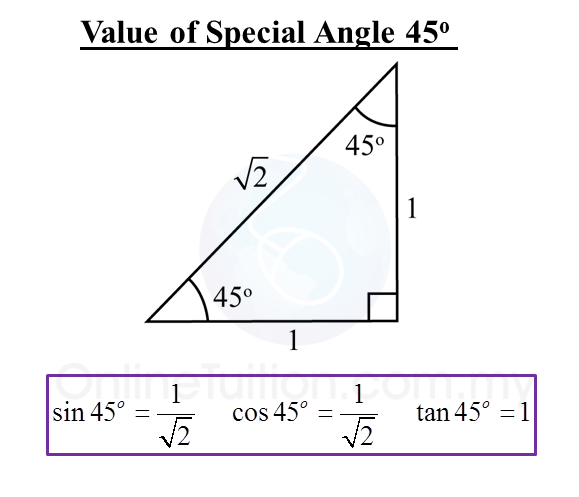2.   1 degree is equal to 60 minutes.
1o= 60’

3.   A scientific calculator can be used to find the value of the sine, cosine or tangent of an angle.
Example:
sin 40.6o = 0.5954

 Calculator Computation     Press [sin] [40.6] [=] 0.595383839
4.   Given the values of sine, cosine and tangent, we can find the angles using a scientific calculator.
Example:
tan x = 1.7862
x = 67o30’

 Calculator Computation Press [shift] [tan][1.7862]  [=][o’’’]  67o30’